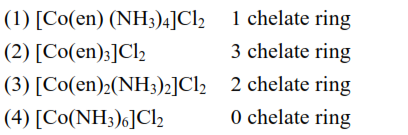# Which one of the following metalQuestion:

Which one of the following metal complexes is most stable?

1. $\left[\mathrm{Co}(\mathrm{en})\left(\mathrm{NH}_{3}\right)_{4}\right] \mathrm{Cl}_{2}$

2. $\left[\mathrm{Co}(\mathrm{en})_{3}\right] \mathrm{Cl}_{2}$

3. $\left[\mathrm{Co}(\mathrm{en})_{2}\left(\mathrm{NH}_{3}\right)_{2}\right] \mathrm{Cl}_{2}$

4. $\left[\mathrm{Co}\left(\mathrm{NH}_{3}\right)_{6}\right] \mathrm{Cl}_{2}$

Correct Option: , 2

Solution:

Complex $\left[\mathrm{Co}(\mathrm{en})_{3}\right] \mathrm{Cl}_{2}$ is most stable complex among the given complex compounds because more number of chelate rings are present in this complex as compare to others.